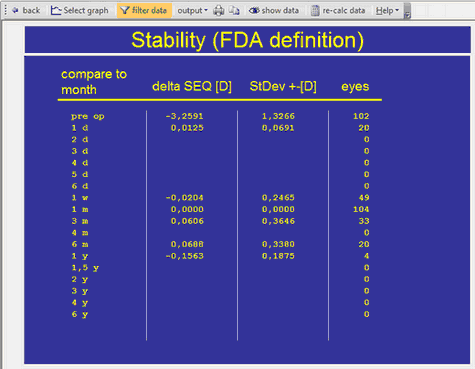﻿ Making Graphs > Refractive Outcome Graphs > Stability (FDA Definition)
 Navigation:  Making Graphs > Refractive Outcome Graphs > Stability (FDA Definition)Stability in between two selected follow up time intervals should only be calculated on paired data,  according to FDA definitions. Only eyes, which have follow up data at both time intervals, will be included.

Below an example where the first time interval is set to 1 month --> values for delta SEQ and StDev are zero. Between 1 month and 1 year a regression of -0.15 D occurred.

Note: there may be discrepancies between the normal stability graph and this table, because only paired data sets are included.